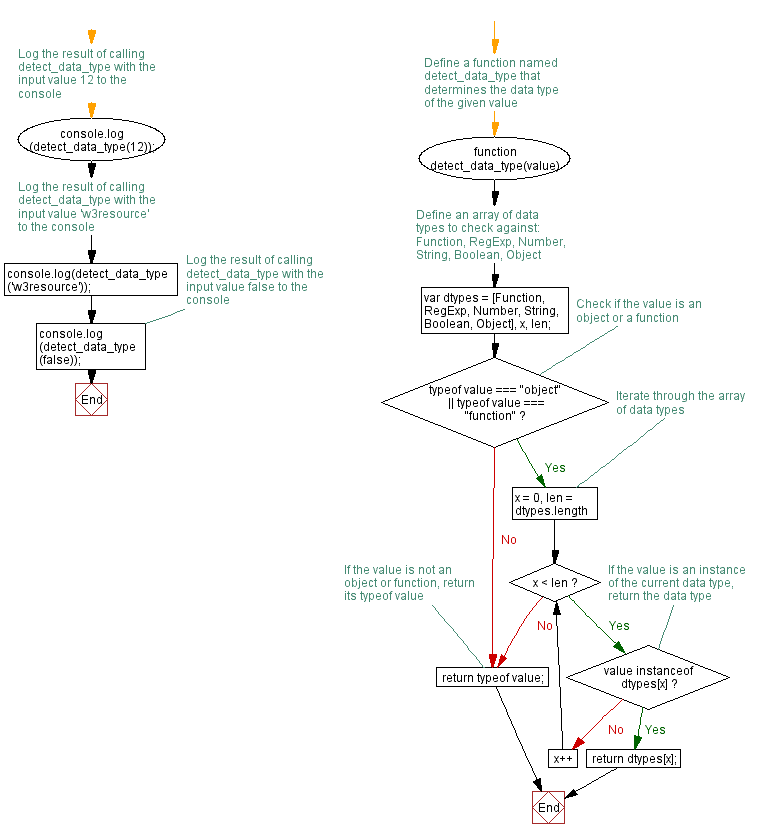# JavaScript: Get the data type

## JavaScript Function: Exercise-9 with Solution

Write a JavaScript function that accepts an argument and returns the type.

Note : There are six possible values that typeof returns: object, boolean, function, number, string, and undefined.

Sample Solution: -

HTML Code:

``````<!DOCTYPE html>
<html>
<meta charset=utf-8 />
<title>Detect JavaScript Data Types</title>
<body>

</body>
</html>
```
```

JavaScript Code:

``````function detect_data_type(value)
{
var dtypes = [Function, RegExp, Number, String, Boolean, Object], x, len;

if (typeof value === "object" || typeof value === "function")
{
for (x = 0, len = dtypes.length; x < len; x++)
{
if (value instanceof dtypes[x])
{
return dtypes[x];
}
}
}

return typeof value;
}
console.log(detect_data_type(12));
console.log(detect_data_type('w3resource'));
console.log(detect_data_type(false));
```
```

Sample Output:

```number
string
boolean
```

Flowchart:Live Demo:

See the Pen JavaScript -Check a number is prime or not-function-ex- 8 by w3resource (@w3resource) on CodePen.

Improve this sample solution and post your code through Disqus

What is the difficulty level of this exercise?

Test your Programming skills with w3resource's quiz.

﻿